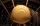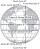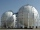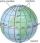Sphere - problems - page 4

1. InscribedCube is inscribed in the cube. Determine its volume if the edge of the cube is 10 cm long.
2. Iron ballThe iron ball has a weight of 100 kilograms. Calculate the volume, radius, and surface if the iron's density is h = 7.6g/cm3.
3. Tropical, mild and arcticHow many percents of the Earth's surface lies in the tropical, mild and arctic range? The border between the ranges is the parallel 23°27 'and 66°33'.
4. Hemispherical hollowThe vessel hemispherical hollow is filled with water to a height of 10 cm =. How many liters of water are inside if the inside diameter of the hollow is d = 28cm?
5. Spherical tankThe tank of a water tower is a sphere of radius 35ft. If the tank is filled to one quarter of full, what is the height of the water?
6. PilotHow high is the airplane's pilot to see 0.001 of Earth's surface?
7. Spherical capPlace a part of the sphere on a 4.6 cm cylinder so that the surface of this section is 20 cm2. Determine the radius r of the sphere from which the spherical cap was cut.
8. Half-sphere roofThe roof above the castle tower has the shape of a 12.8 m diameter half-sphere. What is the cost of this roof, if the cost of 1 square meter is 12 euros and 40 cents?
9. Tangent spheresA sphere with a radius of 1 m is placed in the corner of the room. What is the largest sphere size that fits into the corner behind it? Additional info: Two spheres are placed in a corner of a room. The spheres are each tangent to the walls and floor and
10. Volume of ballFind the volume of a volleyball that has a radius of 4 1/2 decimeters. Use 22/7 for π
11. Earth's circumferenceCalculate the Earth's circumference of the parallel 48 degrees and 10 minutes.

We apologize, but in this category are not a lot of examples.
Do you have an interesting mathematical problem that you can't solve it? Enter it, and we can try to solve it.

To this e-mail address, we will reply solution; solved examples are also published here. Please enter e-mail correctly and check whether you don't have a full mailbox.